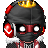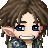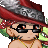## SignatureCharacter profile:
Name: Dante.
Age: ???
Class: Pure blood vampire.
Info: Dante, though being one of the few pure blooded vampires, sometimes becomes too easily flustered. However when given a task he stops at nothing to accomplish his goals.

## Forums

Posts per Day: 0.21

Total Posts: 1298

Latest Posts

## CedantesRegistered: 11/13/2006

Gender: Male

## Contact

• Send Message

Right now im engaged to my amazing fiance who has done so much for me smile to bad it wont last sad cause soon she will be my wife ^-^ and i cant wait ^-^

my all time fave poem ^^

LOVELESS

Prologue
When the war of the beasts brings about the world’s end
The goddess descends from the sky
Wings of light and dark spread afar
She guides us to bliss, her gift everlasting

Act I
Infinite in mystery is the gift of the goddess
We seek it thus, and take it to the sky
Ripples form on the water’s surface
The wandering soul knows no rest.

Act II
There is no hate, only joy
For you are beloved by the goddess
Hero of the dawn, Healer of worlds

Dreams of the morrow hath the shattered soul
Pride is lost
Wings stripped away, the end is nigh

Act III
My friend, do you fly away now?
To a world that abhors you and I?
All that awaits you is a somber morrow
No matter where the winds may blow

Is the bringer of life, the gift of the goddess

Even if the morrow is barren of promises
Nothing shall forestall my return

Act IV
My friend, the fates are cruel
There are no dreams, no honor remains
The arrow has left the bow of the goddess

My soul, corrupted by vengeance
Hath endured torment, to find the end of the journey
In my own salvation

Legend shall speak
Of sacrifice at world’s end
The wind sails over the water’s surface
Quietly, but surely

Act V
Even if the morrow is barren of promises
Nothing shall forestall my return
To become the dew that quenches the land
To spare the sands, the seas, the skies
I offer thee this silent sacrifice

## Friends

View All Friends

••••••••••Report | 11/05/2009 11:37 amstill need 900 gold D:
Report | 11/05/2009 11:36 am:O OMG
Report | 11/05/2009 11:36 am-and again :3
Report | 11/05/2009 11:35 amReport | 11/05/2009 11:34 amDANTE OWNS biggrin
Report | 11/05/2009 11:33 am`^_^ ^_^ ^_^ ^_^ ^_^ ^_^ ^_^ ^_^^_^ ^_^ ^_^ ^_^ ^_^ ^_^ ^_^ ^_^^_^ ^_^ ^_^ ^_^ ^_^ ^_^ ^_^ ^_^^_^ ^_^ ^_^ ^_^ ^_^ ^_^ ^_^ ^_^^_^ ^_^ ^_^ ^_^ ^_^ ^_^ ^_^ ^_^^_^ ^_^ ^_^ ^_^ ^_^ ^_^ ^_^ ^_^^_^ ^_^ ^_^ ^_^ ^_^ ^_^ ^_^ ^_^^_^ ^_^ ^_^ ^_^ ^_^ ^_^ ^_^ ^_^^_^ ^_^ ^_^ ^_^ ^_^ ^_^ ^_^ ^_^^_^ ^_^ ^_^ ^_^ ^_^ ^_^ ^_^ ^_^^_^ ^_^ ^_^ ^_^ ^_^ ^_^ ^_^ ^_^^_^ ^_^ ^_^ ^_^ ^_^ ^_^ ^_^ ^_^^_^ ^_^ ^_^ ^_^ ^_^ ^_^ ^_^ ^_^^_^ ^_^ ^_^ ^_^ ^_^ ^_^ ^_^ ^_^^_^ ^_^ ^_^ ^_^ ^_^ ^_^ ^_^ ^_^^_^ ^_^ ^_^ ^_^ ^_^ ^_^ ^_^ ^_^^_^ ^_^ ^_^ ^_^ ^_^ ^_^ ^_^ ^_^^_^ ^_^ ^_^ ^_^ ^_^ ^_^ ^_^ ^_^^_^ ^_^ ^_^ ^_^ ^_^ ^_^ ^_^ ^_^^_^ ^_^ ^_^ ^_^ ^_^ ^_^ ^_^ ^_^^_^ ^_^ ^_^ ^_^ ^_^ ^_^ ^_^ ^_^^_^ ^_^ ^_^ ^_^ ^_^ ^_^ ^_^ ^_^^_^ ^_^ ^_^ ^_^ ^_^ ^_^ ^_^ ^_^^_^ ^_^ ^_^ ^_^ ^_^ ^_^ ^_^ ^_^^_^ ^_^ ^_^ ^_^ ^_^ ^_^ ^_^ ^_^^_^ ^_^ ^_^ ^_^ ^_^ ^_^ ^_^ ^_^^_^ ^_^ ^_^ ^_^ ^_^ ^_^ ^_^ ^_^^_^ ^_^ ^_^ ^_^ ^_^ ^_^ ^_^ ^_^^_^ ^_^ ^_^ ^_^ ^_^ ^_^ ^_^ ^_^^_^ ^_^ ^_^ ^_^ ^_^ ^_^ ^_^ ^_^^_^ ^_^ ^_^ ^_^ ^_^ ^_^ ^_^ ^_^^_^ ^_^ ^_^ ^_^ ^_^ ^_^ ^_^ ^_^^_^ ^_^ ^_^ ^_^ ^_^ ^_^ ^_^ ^_^^_^ ^_^ ^_^ ^_^ ^_^ ^_^ ^_^ ^_^^_^ ^_^ ^_^ ^_^ ^_^ ^_^ ^_^ ^_^^_^ ^_^ ^_^ ^_^ ^_^ ^_^ ^_^ ^_^^_^ ^_^ ^_^ ^_^ ^_^ ^_^ ^_^ ^_^^_^ ^_^ ^_^ ^_^ ^_^ ^_^ ^_^ ^_^^_^ ^_^ ^_^ ^_^ ^_^ ^_^ ^_^ ^_^^_^ ^_^ ^_^ ^_^ ^_^ ^_^ ^_^ ^_^^_^ ^_^ ^_^ ^_^ ^_^ ^_^ ^_^ ^_^^_^ ^_^ ^_^ ^_^ ^_^ ^_^ ^_^ ^_^^_^ ^_^ ^_^ ^_^ ^_^ ^_^ ^_^ ^_^^_^ ^_^ ^_^ ^_^ ^_^ ^_^ ^_^ ^_^^_^ ^_^ ^_^ ^_^ ^_^ ^_^ ^_^ ^_^^_^ ^_^ ^_^ ^_^ ^_^ ^_^ ^_^ ^_^^_^ ^_^ ^_^ ^_^ ^_^ ^_^ ^_^ ^_^^_^ ^_^ ^_^ ^_^ ^_^ ^_^ ^_^ ^_^^_^ ^_^ ^_^ ^_^ ^_^ ^_^ ^_^ ^_^^_^ ^_^ ^_^ ^_^ ^_^ ^_^ ^_^ ^_^^_^ ^_^ ^_^ ^_^ ^_^ ^_^ ^_^ ^_^^_^ ^_^ ^_^ ^_^ ^_^ ^_^ ^_^ ^_^^_^ ^_^ ^_^ ^_^ ^_^ ^_^ ^_^ ^_^^_^ ^_^ ^_^ ^_^ ^_^ ^_^ ^_^ ^_^^_^ ^_^ ^_^ ^_^ ^_^ ^_^ ^_^ ^_^^_^ ^_^ ^_^ ^_^ ^_^ ^_^ ^_^ ^_^^_^ ^_^ ^_^ ^_^ ^_^ ^_^ ^_^ ^_^^_^ ^_^ ^_^ ^_^ ^_^ ^_^ ^_^ ^_^^_^ ^_^ ^_^ ^_^ ^_^ ^_^ ^_^ ^_^^_^ ^_^ ^_^ ^_^ ^_^ ^_^ ^_^ ^_^^_^ ^_^ ^_^ ^_^ ^_^ ^_^ ^_^ ^_^^_^ ^_^ ^_^ ^_^ ^_^ ^_^ ^_^ ^_^^_^ ^_^ ^_^ ^_^ ^_^ ^_^ ^_^ ^_^^_^ ^_^ ^_^ ^_^ ^_^ ^_^ ^_^ ^_^^_^ ^_^ ^_^ ^_^ ^_^ ^_^ ^_^ ^_^^_^ ^_^ ^_^ ^_^ ^_^ ^_^ ^_^ ^_^^_^ ^_^ ^_^ ^_^ ^_^ ^_^ ^_^ ^_^^_^ ^_^ ^_^ ^_^ ^_^ ^_^ ^_^ ^_^^_^ ^_^ ^_^ ^_^ ^_^ ^_^ ^_^ ^_^^_^ ^_^ ^_^ ^_^ ^_^ ^_^ ^_^ ^_^^_^ ^_^ ^_^ ^_^ ^_^ ^_^ ^_^ ^_^^_^ ^_^ ^_^ ^_^ ^_^ ^_^ ^_^ ^_^^_^ ^_^ ^_^ ^_^ ^_^ ^_^ ^_^ ^_^^_^ ^_^ ^_^ ^_^ ^_^ ^_^ ^_^ ^_^^_^ ^_^ ^_^ ^_^ ^_^ ^_^ ^_^ ^_^^_^ ^_^ ^_^ ^_^ ^_^ ^_^ ^_^ ^_^^_^ ^_^ ^_^ ^_^ ^_^ ^_^ ^_^ ^_^^_^ ^_^ ^_^ ^_^ ^_^ ^_^ ^_^ ^_^^_^ ^_^ ^_^ ^_^ ^_^ ^_^ ^_^ ^_^^_^ ^_^ ^_^ ^_^ ^_^ ^_^ ^_^ ^_^^_^ ^_^ ^_^ ^_^ ^_^ ^_^ ^_^ ^_^^_^ ^_^ ^_^ ^_^ ^_^ ^_^ ^_^ ^_^^_^ ^_^ ^_^ ^_^ ^_^ ^_^ ^_^ ^_^^_^ ^_^ ^_^ ^_^ ^_^ ^_^ ^_^ ^_^^_^ ^_^ ^_^ ^_^ ^_^ ^_^ ^_^ ^_^^_^ ^_^ ^_^ ^_^ ^_^ ^_^ ^_^ ^_^^_^ ^_^ ^_^ ^_^ ^_^ ^_^ ^_^ ^_^^_^ ^_^ ^_^ ^_^ ^_^ ^_^ ^_^ ^_^^_^ ^_^ ^_^ ^_^ ^_^ ^_^ ^_^ ^_^^_^ ^_^ ^_^ ^_^ ^_^ ^_^ ^_^ ^_^^_^ ^_^ ^_^ ^_^ ^_^ ^_^ ^_^ ^_^^_^ ^_^ ^_^ ^_^ ^_^ ^_^ ^_^ ^_^^_^ ^_^ ^_^ ^_^ ^_^ ^_^ ^_^ ^_^^_^ ^_^ ^_^ ^_^ ^_^ ^_^ ^_^ ^_^^_^ ^_^ ^_^ ^_^ ^_^ ^_^ ^_^ ^_^^_^ ^_^ ^_^ ^_^ ^_^ ^_^ ^_^ ^_^^_^ ^_^ ^_^ ^_^ ^_^ ^_^ ^_^ ^_^^_^ ^_^ ^_^ ^_^ ^_^ ^_^ ^_^ ^_^^_^ ^_^ ^_^ ^_^ ^_^ ^_^ ^_^ ^_^^_^ ^_^ ^_^ ^_^ ^_^ ^_^ ^_^ ^_^^_^ ^_^ ^_^ ^_^ ^_^ ^_^ ^_^ ^_^^_^ ^_^ ^_^ ^_^ ^_^ ^_^ ^_^ ^_^^_^ ^_^ ^_^ ^_^ ^_^ ^_^ ^_^ ^_^^_^ ^_^ ^_^ ^_^ ^_^ ^_^ ^_^ ^_^^_^ ^_^ ^_^ ^_^ ^_^ ^_^ ^_^ ^_^^_^ ^_^ ^_^ ^_^ ^_^ ^_^ ^_^ ^_^^_^ ^_^ ^_^ ^_^ ^_^ ^_^ ^_^ ^_^^_^ ^_^ ^_^ ^_^ ^_^ ^_^ ^_^ ^_^^_^ ^_^ ^_^ ^_^ ^_^ ^_^ ^_^ ^_^^_^ ^_^ ^_^ ^_^ ^_^ ^_^ ^_^ ^_^^_^ ^_^ ^_^ ^_^ ^_^ ^_^ ^_^ ^_^^_^ ^_^ ^_^ ^_^ ^_^ ^_^ ^_^ ^_^^_^ ^_^ ^_^ ^_^ ^_^ ^_^ ^_^ ^_^^_^ ^_^ ^_^ ^_^ ^_^ ^_^ ^_^ ^_^^_^ ^_^ ^_^ ^_^ ^_^ ^_^ ^_^ ^_^^_^ ^_^ ^_^ ^_^ ^_^ ^_^ ^_^ ^_^^_^ ^_^ ^_^ ^_^ ^_^ ^_^ ^_^ ^_^^_^ ^_^ ^_^ ^_^ ^_^ ^_^ ^_^ ^_^^_^ ^_^ ^_^ ^_^ ^_^ ^_^ ^_^ ^_^^_^ ^_^ ^_^ ^_^ ^_^ ^_^ ^_^ ^_^^_^ ^_^ ^_^ ^_^ ^_^ ^_^ ^_^ ^_^^_^ ^_^ ^_^ ^_^ ^_^ ^_^ ^_^ ^_^^_^ ^_^ ^_^ ^_^ ^_^ ^_^ ^_^ ^_^^_^ ^_^ ^_^ ^_^ ^_^ ^_^ ^_^ ^_^^_^ ^_^ ^_^ ^_^ ^_^ ^_^ ^_^ ^_^^_^ ^_^ ^_^ ^_^ ^_^ ^_^ ^_^ ^_^^_^ ^_^ ^_^ ^_^ ^_^ ^_^ ^_^ ^_^^_^ ^_^ ^_^ ^_^ ^_^ ^_^ ^_^ ^_^^_^ ^_^ ^_^ ^_^ ^_^ ^_^ ^_^ ^_^^`
Report | 11/05/2009 11:32 amLAAAAAAAAAAAAAAAAAAAAAAAAAAAAAAAAAAAAAAALLLLLLLLLLLLLAAAAAAAAAAAAAAAAAAAAAAAAAAAAAAAAAAAAAAAAAAAAAAAAAAAAAAAAAAAAAAAAAAAAAAAAAAAAAAAAAAAAAAAAAAAAAAAAAAAAAAAAAAAAAAAAAAAAAAAAAAAAAA

^ ^
_
Report | 11/05/2009 11:31 amBUMMMMMMMMMMMMMMMMMMMMMMMMMMMMMMMMMMMMMMMMMMMMMMMMM
Report | 11/05/2009 11:30 amSEXYYYYYYYYYYYYYYYYYYYYYYYYYYYYYYY
biggrin
Report | 10/27/2009 4:51 pm